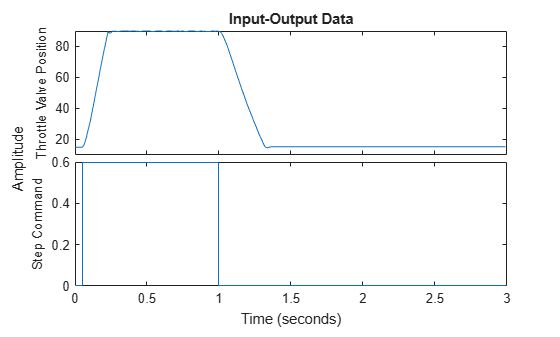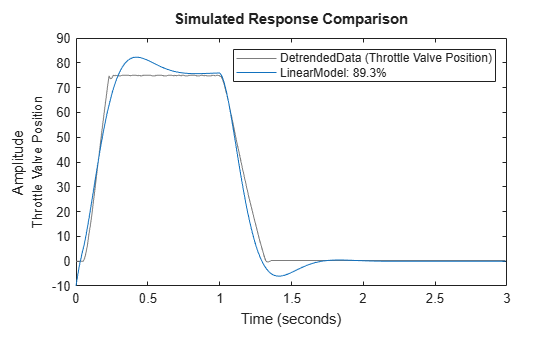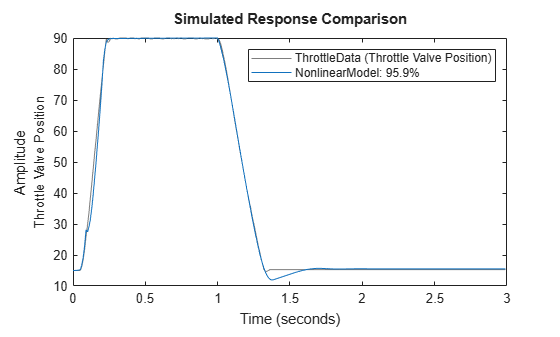# Estimate Nonlinear ARX Models Initialized Using Linear ARX Models

This example shows how to estimate nonlinear ARX models by using linear ARX models.

`load throttledata.mat`

This command loads the data object `ThrottleData` into the workspace. The object contains input and output samples collected from an engine throttle system, sampled at a rate of 100 Hz.

A DC motor controls the opening angle of the butterfly valve in the throttle system. A step signal (in volts) drives the DC motor. The output is the angular position (in degrees) of the valve.

Plot the data to view and analyze the data characteristics.

`plot(ThrottleData)`In the normal operating range of 15-90 degrees, the input and output variables have a linear relationship. You use a linear model of low order to model this relationship.

In the throttle system, a hard stop limits the valve position to `90` degrees, and a spring brings the valve to `15` degrees when the DC motor is turned off. These physical components introduce nonlinearities that a linear model cannot capture.

Estimate an ARX model to model the linear behavior of this single-input single-output system in the normal operating range.

Detrend the data because linear models cannot capture offsets.

```Tr = getTrend(ThrottleData); Tr.OutputOffset = 15; DetrendedData = detrend(ThrottleData,Tr);```

Estimate a linear ARX model with na=2, nb=1, nk=1.

```opt = arxOptions('Focus','simulation'); LinearModel = arx(DetrendedData,[2 1 1],opt);```

Compare the simulated model response with the estimation data.

`compare(DetrendedData, LinearModel)`The linear model captures the rising and settling behavior in the linear operating range but does not account for output saturation at 90 degrees.

Estimate a nonlinear ARX model to model the output saturation.

```optNL = nlarxOptions('Focus','simulation'); NonlinearModel = nlarx(ThrottleData,LinearModel,'idSigmoidNetwork',optNL);```

The software uses the orders and delay of the linear model for the orders of the nonlinear model. In addition, the software computes the linear function of `sigmoidnet` nonlinearity estimator.

Compare the nonlinear model with the estimation data.

`compare(ThrottleData, NonlinearModel)`The model captures the nonlinear effects (output saturation) and improves the overall fit to data.

## SupportGet trial now